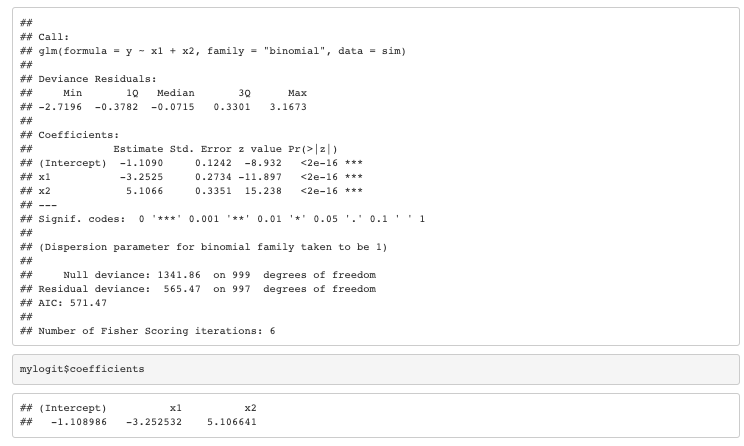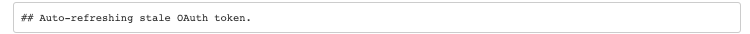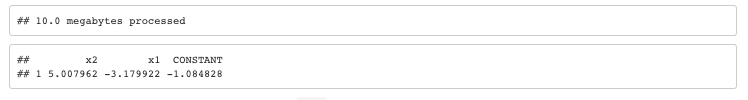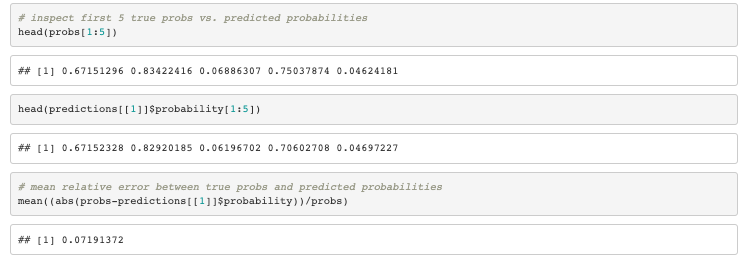# In-Database Logistic Regression with R

by Roland Stevenson

Roland Stevenson is a data scientist and consultant who may be reached on Linkedin.

In a previous article we illustrated how to calculate xgboost model predictions in-database. This was referenced and incorporated into tidypredict. After learning more about what the tidypredict team is up to, I discovered another tidyverse package called modeldb that fits models in-database. It currently supports linear regression and k-means clustering, so I thought I would provide an example of how to do in-database logistic regression.

Rather than focusing on the details of logistic regression, we will focus more on how we can use R and some carefully written SQL statements to iteratively minimize a cost function. We will also use the condusco R package, which allows us to iterate through the results of a query easily.

## A Simple Logistic Regression Example

Let’s start with a simple logistic regression example. We’ll simulate an outcome $$y$$ based on the fact that $$Pr(y=1) = \frac{e^{\beta x}}{1+e^{\beta x}}$$. Here $$\beta$$ is a vector containing the coefficients we will later be estimating (including an intercept term). In the example below, our $$x$$ values are uniform random values between -1 and 1.

set.seed(1)

# the number of samples
n <- 1000

# uniform random on (-1,1)
x1 <- 2*runif(n)-1
x2 <- 2*runif(n)-1
x <- cbind(1, x1, x2)

# our betas
beta <- c(-1, -3.0, 5.0)

probs <- exp(beta %*% t(x))/(1+exp(beta %*% t(x)))

y <- rbinom(n,1,probs)

sim <- data.frame(id = seq(1:n), y = y, x1 = x1, x2 = x2)

mylogit <- glm(y ~ x1 + x2, data = sim, family = "binomial")
summary(mylogit)
mylogit$coefficientsAs expected, the coefficients of our logistic model successfully approximate the parameters in our beta vector. ## In-database Logistic Regression Now, let’s see if we can find a way to calculate these same coefficients in-database. In this example, we’re going to use Google BigQuery as our database, and we’ll use condusco’s run_pipeline_gbq function to iteratively run the functions we define later on. To do this, we’ll need to take care of some initial housekeeping: library(bigrquery) library(whisker) library(condusco) # Uncomment and define your own config # config <- list( # project = '<YOUR GBQ PROJECT>', # dataset = '<YOUR GBQ DATASET>', # table_prefix = '<A TABLE_PREFIX TO USE>' # ) # a simple whisker.render helper function for our use-case wr <- function(s, params=config){whisker.render(s,params)} # put the simulated data in GBQ insert_upload_job( project = wr('{{{project}}}'), dataset = wr('{{{dataset}}}'), table = "logreg_sim", values = sim, write_disposition = "WRITE_TRUNCATE" )Now, we’ll create the pipelines to do the logistic regression. Please note that the code below is quite verbose. While all of it is needed for the code to work, we’ll just focus on understanding how a couple of steps work. Once we understand one step, the rest is pretty easy. Feel free to skip ahead. First, we create a pipeline that does two things: • create a main table containing all of our global settings • calls another pipeline (log_reg_stack) with the global settings as inputs Importantly, note that all of the parameters (eg. {{{project}}}) are dynamically swapped out in the query below with the wr function and the params variables. So this pipeline dynamically creates a query based on the parameters passed to it. We will call this pipeline later to run the process. # # Pipeline: log_reg # log_reg <- function(params){ print ("log_reg") query <- ' CREATE OR REPLACE TABLE {{{dataset}}}.{{{table_prefix}}}_settings AS SELECT "{{{project}}}" AS project, "{{{dataset}}}" AS dataset, "{{{data_table}}}" AS data_table, {{{max_steps}}} AS max_steps, {{{error_tol}}} AS error_tol, {{{learning_rate}}} AS learning_rate, "{{{id_column}}}" AS id_column, "{{{label_column}}}" AS label_column, "{{{fieldnames}}}" AS fieldnames, "{{{constant_id}}}" AS constant_id, "{{{table_prefix}}}" AS table_prefix ' query_exec( project = wr('{{{project}}}', params), query = wr(query, params), use_legacy_sql = FALSE ) # Now run the log_reg_stack pipeline and pass the settings to it invocation_query <- ' SELECT * FROM {{{dataset}}}.{{table_prefix}}_settings ' run_pipeline_gbq( log_reg_stack, wr(invocation_query, params), wr('{{{project}}}', params), use_legacy_sql = FALSE ) } The above pipeline calls another pipeline, log_reg_stack, which is defined below. log_reg_stack creates a table with the field names that we will use in the logistic regression and then runs log_reg_stack_field on each of the field names. Note that the invocation_query below contains a query that results in one or more rows containing a field name. run_pipeline_gbq takes the results and iterates over them, calling log_reg_stack_field on each one. Finally, it creates the _labels table and calls log_reg_setup, passing it the results of the global settings query. # # Pipeline: stack variables # log_reg_stack <- function(params){ print ("log_reg_stack") # Table: _fieldnames query <- " CREATE OR REPLACE TABLE {{{dataset}}}.{{{table_prefix}}}_fieldnames AS SELECT TRIM(fieldname) AS fieldname FROM ( SELECT split(fieldnames,',') AS fieldname FROM ( SELECT '{{{fieldnames}}}' AS fieldnames ) ), UNNEST(fieldname) as fieldname GROUP BY 1 " query_exec( project = wr('{{{project}}}', params), query = wr(query, params), use_legacy_sql = FALSE ) # Run _stack_field query <- " DROP TABLE IF EXISTS {{{dataset}}}.{{{table_prefix}}}_stacked " tryCatch({ query_exec( project = wr('{{{project}}}', params), query = wr(query, params), use_legacy_sql = FALSE )}, error = function(e){ print(e) }) invocation_query <- " SELECT a.fieldname AS fieldname, b.* FROM ( SELECT fieldname FROM {{{dataset}}}.{{{table_prefix}}}_fieldnames GROUP BY fieldname ) a CROSS JOIN ( SELECT * FROM {{{dataset}}}.{{{table_prefix}}}_settings ) b " run_pipeline_gbq( log_reg_stack_field, wr(invocation_query, params), wr('{{{project}}}', params), use_legacy_sql = FALSE ) # Table: _labels query <- " CREATE OR REPLACE TABLE {{{dataset}}}.{{{table_prefix}}}_labels AS SELECT {{{id_column}}} AS id, {{{label_column}}} AS label FROM {{{data_table}}} " query_exec( project = wr('{{{project}}}', params), query = wr(query, params), use_legacy_sql = FALSE ) # Run _setup invocation_query <- " SELECT * FROM {{{dataset}}}.{{{table_prefix}}}_settings " run_pipeline_gbq( log_reg_setup, wr(invocation_query, params), wr('{{{project}}}', params), use_legacy_sql = FALSE ) } The log_reg_stack_field and log_reg_setup pipelines are not particularly interesting. They do the groundwork needed to allow the log_reg_loop pipeline to iterate. The _stacked table contains the feature names and their values, and the _feature_stats and features_stacked_vni tables contains normalized values used later. Finally, the _fit_params table contains the value of the fit parameters that will be updated as we iteratively minimize the cost function in the loop. The log_reg_setup pipeline ends by calling log_reg_loop, passing it the results of the global settings query. log_reg_stack_field <- function(params){ print ("log_reg_stack_field") destination_table <- '{{{dataset}}}.{{{table_prefix}}}_stacked' query <- " SELECT {{{id_column}}} AS id, LTRIM('{{{fieldname}}}') AS feature_name, CAST({{{fieldname}}} AS FLOAT64) AS vi FROM {{{data_table}}} " query_exec( project = wr('{{{project}}}', params), query = wr(query, params), destination_table = wr(destination_table, params), use_legacy_sql = FALSE, write_disposition = 'WRITE_APPEND', create_disposition = 'CREATE_IF_NEEDED' ) } log_reg_setup <- function(params){ print ("log_reg_setup") query <- " CREATE OR REPLACE TABLE {{{dataset}}}.{{{table_prefix}}}_feature_stats AS SELECT feature_name, AVG(vi) AS mean, STDDEV(vi) AS stddev FROM {{{dataset}}}.{{{table_prefix}}}_stacked GROUP BY feature_name " query_exec( project = wr('{{{project}}}', params), query = wr(query, params), use_legacy_sql = FALSE ) query <- " CREATE OR REPLACE TABLE {{{dataset}}}.{{{table_prefix}}}_features_stacked_vni AS SELECT a.id AS id, a.feature_name AS feature_name, CASE WHEN b.stddev > 0.0 THEN (vi - b.mean) / b.stddev ELSE vi - b.mean END AS vni FROM {{{dataset}}}.{{{table_prefix}}}_stacked a JOIN {{{dataset}}}.{{{table_prefix}}}_feature_stats b ON a.feature_name = b.feature_name " query_exec( project = wr('{{{project}}}', params), query = wr(query, params), use_legacy_sql = FALSE ) query <- " INSERT INTO {{{dataset}}}.{{{table_prefix}}}_features_stacked_vni (id, feature_name, vni) SELECT id, '{{{constant_id}}}' as feature_name, 1.0 as vni FROM {{{dataset}}}.{{{table_prefix}}}_stacked GROUP BY 1,2,3 " query_exec( project = wr('{{{project}}}', params), query = wr(query, params), use_legacy_sql = FALSE ) query <- " CREATE OR REPLACE TABLE {{{dataset}}}.{{{table_prefix}}}_fit_params AS SELECT step, param_id, param_value, cost, stop, message FROM ( SELECT 1 as step, feature_name as param_id, 0.0 as param_value, 1e6 as cost, false as stop, '' as message FROM {{{dataset}}}.{{{table_prefix}}}_stacked GROUP BY param_id ) UNION ALL ( SELECT 1 as step, '{{{constant_id}}}' as param_id, 0.0 as param_value, 1e6 as cost, false as stop, '' as message ) " query_exec( project = wr('{{{project}}}', params), query = wr(query, params), use_legacy_sql = FALSE ) # Run _loop invocation_query <- " SELECT * FROM {{{dataset}}}.{{{table_prefix}}}_settings " run_pipeline_gbq( log_reg_loop, wr(invocation_query, params), wr('{{{project}}}', params), use_legacy_sql = FALSE ) } Next, we’ll create a loop pipeline that will iteratively calculate the cost function and update the _fit_params table with the latest update. # # Pipeline: loop # log_reg_loop <- function(params){ print ("log_reg_loop") query <- " CREATE OR REPLACE TABLE {{{dataset}}}.{{{table_prefix}}}_x_dot_beta_i AS SELECT a.id AS id, SUM(a.vni * b.param_value) AS x_dot_beta_i FROM {{{dataset}}}.{{{table_prefix}}}_features_stacked_vni a RIGHT JOIN ( SELECT param_id, param_value FROM {{{dataset}}}.{{{table_prefix}}}_fit_params WHERE STEP = (SELECT max(step) FROM {{{dataset}}}.{{{table_prefix}}}_fit_params) ) b ON a.feature_name = b.param_id GROUP BY 1 " query_exec( project = wr('{{{project}}}', params), query = wr(query, params), use_legacy_sql = FALSE ) query <- ' INSERT INTO {{{dataset}}}.{{{table_prefix}}}_fit_params (step, param_id, param_value, cost, stop, message) SELECT b.step + 1 as step, b.param_id as param_id, b.param_value - {{{learning_rate}}} * err as param_value, -1.0 * a.cost as cost, CASE WHEN ( abs((b.cost-(-1.0*a.cost))/b.cost) < {{{error_tol}}} ) OR (step+1 > {{{max_steps}}}) THEN true ELSE false END AS stop, CONCAT( "cost: ", CAST(abs((b.cost-(-1.0*a.cost))/b.cost) AS STRING), " error_tol: ", CAST({{{error_tol}}} AS STRING)) as message FROM ( SELECT param_id, avg(err) as err, avg(cost) as cost FROM ( SELECT a.id, param_id, (1.0/(1.0 + EXP(-1.0 * (c.x_dot_beta_i))) - CAST(label AS FLOAT64)) * vni as err, CAST(label AS FLOAT64) * LOG( 1.0/(1.0 + EXP(-1.0 * (c.x_dot_beta_i))) ) + (1.0-CAST(label AS FLOAT64))*(log(1.0 - (1.0/(1.0 + EXP(-1.0 * (c.x_dot_beta_i)))))) as cost FROM ( SELECT a.id as id, b.param_id as param_id, a.vni as vni, b.param_value as param_value FROM {{{dataset}}}.{{{table_prefix}}}_features_stacked_vni a JOIN ( SELECT param_id, param_value FROM {{{dataset}}}.{{{table_prefix}}}_fit_params WHERE STEP = (SELECT max(step) FROM {{{dataset}}}.{{{table_prefix}}}_fit_params) ) b ON a.feature_name = b.param_id GROUP BY 1,2,3,4 ) a JOIN {{{dataset}}}.{{{table_prefix}}}_labels b ON a.id = b.id JOIN {{{dataset}}}.{{{table_prefix}}}_x_dot_beta_i c ON a.id = c.id ) GROUP BY param_id ) a JOIN ( SELECT * FROM {{{dataset}}}.{{{table_prefix}}}_fit_params WHERE STEP = (SELECT max(step) FROM {{{dataset}}}.{{{table_prefix}}}_fit_params) ) b ON a.param_id = b.param_id ' query_exec( project = wr('{{{project}}}', params), query = wr(query, params), use_legacy_sql = FALSE ) # Loop or stop query <- " SELECT stop AS stop FROM ( SELECT * FROM {{{dataset}}}.{{{table_prefix}}}_fit_params ORDER BY step DESC LIMIT 1 ) " res <- query_exec( wr(query, params), wr('{{{project}}}', params), use_legacy_sql = FALSE ) if(res$stop == FALSE){
print("stop == FALSE")
invocation_query <- '
SELECT *
FROM {{{dataset}}}.{{table_prefix}}_settings
'
run_pipeline_gbq(
log_reg_loop,
wr(invocation_query,  params),
wr('{{{project}}}', params),
use_legacy_sql = FALSE
)
}
else {
print("stop == TRUE")
invocation_query <- '
SELECT *
FROM {{{dataset}}}.{{table_prefix}}_settings
'
run_pipeline_gbq(
log_reg_done,
wr(invocation_query,  params),
wr('{{{project}}}', params),
use_legacy_sql = FALSE
)
}

}

And finally, a log_reg_done pipeline that outputs the results:

#
# Pipeline: done
#
log_reg_done <- function(params){

print ("log_reg_done")

# Display results in norm'd coords
query <- '
SELECT "normalized coords parameters" as message,
step,
param_id,
param_value
FROM {{{dataset}}}.{{{table_prefix}}}_fit_params
WHERE step = (SELECT max(step) from {{{dataset}}}.{{{table_prefix}}}_fit_params)
'

res <- query_exec(
wr(query, params),
wr('{{{project}}}', params),
use_legacy_sql = FALSE
)

print(res)

# Display results in original coords
query <- "
CREATE OR REPLACE TABLE {{{dataset}}}.{{{table_prefix}}}_model_params_stacked
AS
SELECT
param_id,
param_value_rescaled
FROM (
SELECT
a.param_id AS param_id,
a.param_value + b.constant_offset AS param_value_rescaled
FROM (
SELECT
step,
param_id,
param_value
FROM {{{dataset}}}.{{{table_prefix}}}_fit_params
WHERE step = (SELECT max(step) from {{{dataset}}}.{{{table_prefix}}}_fit_params)
AND param_id = 'CONSTANT'
) a
JOIN (
SELECT
step,
'CONSTANT' as param_id,
sum(-1.0*param_value*mean/stddev) as constant_offset
FROM {{{dataset}}}.{{{table_prefix}}}_fit_params a
JOIN {{{dataset}}}.{{{table_prefix}}}_feature_stats b
ON a.param_id = b.feature_name
WHERE step = (SELECT max(step) FROM {{{dataset}}}.{{{table_prefix}}}_fit_params)
GROUP BY 1,2
) b
ON a.param_id = b.param_id
) UNION ALL (
SELECT
param_id,
param_value/stddev as param_value_rescaled
FROM {{{dataset}}}.{{{table_prefix}}}_fit_params a
JOIN {{{dataset}}}.{{{table_prefix}}}_feature_stats b
ON a.param_id = b.feature_name
WHERE step = (SELECT max(step) FROM {{{dataset}}}.{{{table_prefix}}}_fit_params)
GROUP BY 1,2
)
"

res <- query_exec(
wr(query, params),
wr('{{{project}}}', params),
use_legacy_sql = FALSE
)

print(res)

# transpose the _model_params_stacked table
invocation_query <- '
SELECT
a.list,
b.*
FROM (
SELECT CONCAT("[", STRING_AGG(CONCAT("{\\"val\\": \\"",TRIM(fieldname), "\\"}")), "]") AS list
FROM rstevenson.indb_logreg_001_fieldnames
) a
CROSS JOIN (
SELECT *
FROM rstevenson.indb_logreg_001_settings
) b
'

run_pipeline_gbq(
log_reg_model_params,
wr(invocation_query, config),
wr('{{{project}}}', config),
use_legacy_sql = FALSE
)

print("DONE")

}

Our last pipeline, called at the end of the above pipeline, will transpose the stacked model params. In other words, it will output the parameters of the model in separate columns:

log_reg_model_params <- function(params){

query <- "
CREATE OR REPLACE TABLE {{{dataset}}}.{{{table_prefix}}}_model_params
AS
SELECT
{{#list}}
MAX(CASE WHEN param_id='{{val}}' THEN param_value_rescaled END ) AS {{val}},
{{/list}}
MAX(CASE WHEN param_id='{{constant_id}}' THEN param_value_rescaled END ) AS {{constant_id}}
FROM {{{dataset}}}.{{{table_prefix}}}_model_params_stacked
;"

res <- query_exec(
wr(query, params),
wr('{{{project}}}', params),
use_legacy_sql = FALSE
)

print(res)
}

## Running the pipeline

We are now ready to run the log_reg pipeline. We’ll set up the invocation query with all of our global parameters. These will be stored in the _settings table and then, after stacking and setup, the pipeline will iterate through the loop to calculate the logistic regression coefficients.

# Run the log_reg pipeline with the following params (2D test)
invocation_query <- '
SELECT
"{{{project}}}" as project,
"{{{dataset}}}" as dataset,
"{{{table_prefix}}}" as table_prefix,
"{{{dataset}}}.logreg_sim" as data_table,
"25"  as max_steps,
"1e-6" as error_tol,
"6.0"  as learning_rate,
"id"   as id_column,
"y"  as label_column,
"x1, x2"  as fieldnames,
"CONSTANT" as constant_id
'

cat(wr(invocation_query, config))

query_exec(wr(invocation_query, config), project=config$project, use_legacy_sql = FALSE) run_pipeline_gbq( log_reg, wr(invocation_query, config), project = wr('{{{project}}}', config), use_legacy_sql = FALSE ) After running the above, we should be able to query the table that holds the fitted parameters:  query <- " SELECT * FROM {{{dataset}}}.{{{table_prefix}}}_model_params ;" query_exec( wr(query), wr('{{{project}}}'), use_legacy_sql = FALSE )As expected, these results are pretty close to our original beta values. Please keep in mind that this is not ready to be released into the wild. Further improvements include modifications to deal with categorical variables, output describing whether a logistic fit is statistically significant for a particular parameter, and options for controlling step-sizes. But it does show the concept of how an iterative process like logistic regression can be done while using the database to maintain state. ## Prediction Now that we have fit the logistic regression model and the model is stored in the database, we can predict values using the model. We just need a prediction pipeline: # # Pipeline: predict # log_reg_predict <- function(params){ query <- ' SELECT 1/(1+exp(-1.0*(CONSTANT + {{#list}}a.{{val}}*b.{{val}} + {{/list}} + 0))) as probability FROM {{{dataset}}}.{{{table_prefix}}}_model_params a CROSS JOIN {{{data_table}}} b ORDER BY {{{id_column}}} ' res <- query_exec( wr(query, params), wr('{{{project}}}', params), use_legacy_sql = FALSE ) } Note that the above uses whisker to calculate the dot product $$x\beta$$ by expanding a JSON-formatted array of field names into {{#list}}a.{{val}}*b.{{val}} + {{/list}} code. In the code below, we will create a JSON-formatted array of field names. Now let’s run the predictions: # Run the prediction pipeline with the following params invocation_query <- ' SELECT "{{{project}}}" as project, "{{{dataset}}}" as dataset, "{{{table_prefix}}}" as table_prefix, "{{{dataset}}}.logreg_sim" as data_table, "id" as id_column, CONCAT("[", STRING_AGG(CONCAT("{\\"val\\": \\"",TRIM(fieldname), "\\"}")), "]") AS list FROM {{{dataset}}}.{{{table_prefix}}}_fieldnames ' predictions <- run_pipeline_gbq( log_reg_predict, wr(invocation_query, config), project = wr('{{{project}}}', config), use_legacy_sql = FALSE ) Let’s test the rounded predictions to see how well they approximate the outcomes: # inspect first 5 true probs vs. predicted probabilities head(probs[1:5]) head(predictions[]$probability[1:5])

# mean relative error between true probs and predicted probabilities
mean((abs(probs-predictions[]\$probability))/probs)Our model-based logistic regression model predicts the true probabilities with a mean relative error of about 7%.

## Next steps

We have shown how to train and store a logistic regression model in a database. We can then predict outcomes given features that are also stored in the database without having to move data back and forth to a prediction server. In this particular example, it would likely be much faster to move the data to a computer and run the predictions there. However, certain use cases exist where in-database modeling could be an avenue for consideration. Further, since logistic models are fundamental to many types of tree and forest predictors, in-database logistic regression would be a necessary step in developing in-database tree methods. It remains to be seen if this approach can be easily translated into the tidyverse modeldb package.